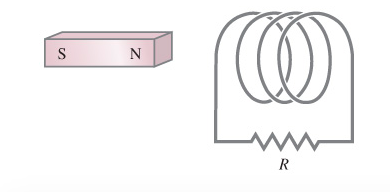# Problem: The north pole of the magnet in the figure is being inserted into the coil.In which direction is the induced current flowing through the resistor R? (Figure 1)A. from right to leftB. from left to right

###### FREE Expert Solution

Magnetic flux:

$\overline{){{\mathbf{\varphi }}}_{{\mathbf{B}}}{\mathbf{=}}{\mathbf{B}}{\mathbf{A}}{\mathbf{c}}{\mathbf{o}}{\mathbf{s}}{\mathbf{\theta }}}$ where θ is the angle between B and A.

In this problem, we are going to apply Lenz's law to get the direction of the induced current.

Lenz's law: The induced electric current flows in a direction such that the current opposes the change in magnetic flux inducing it.

80% (283 ratings)###### Problem Details

The north pole of the magnet in the figure is being inserted into the coil.In which direction is the induced current flowing through the resistor R? (Figure 1)

A. from right to left

B. from left to right